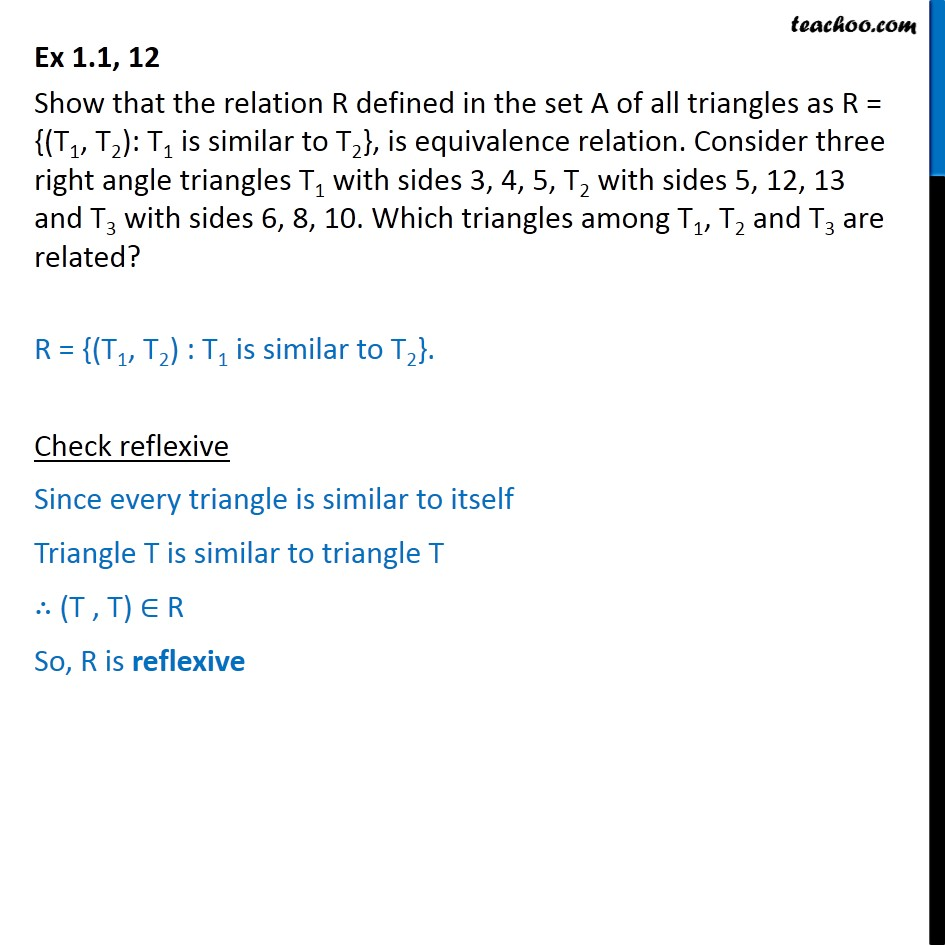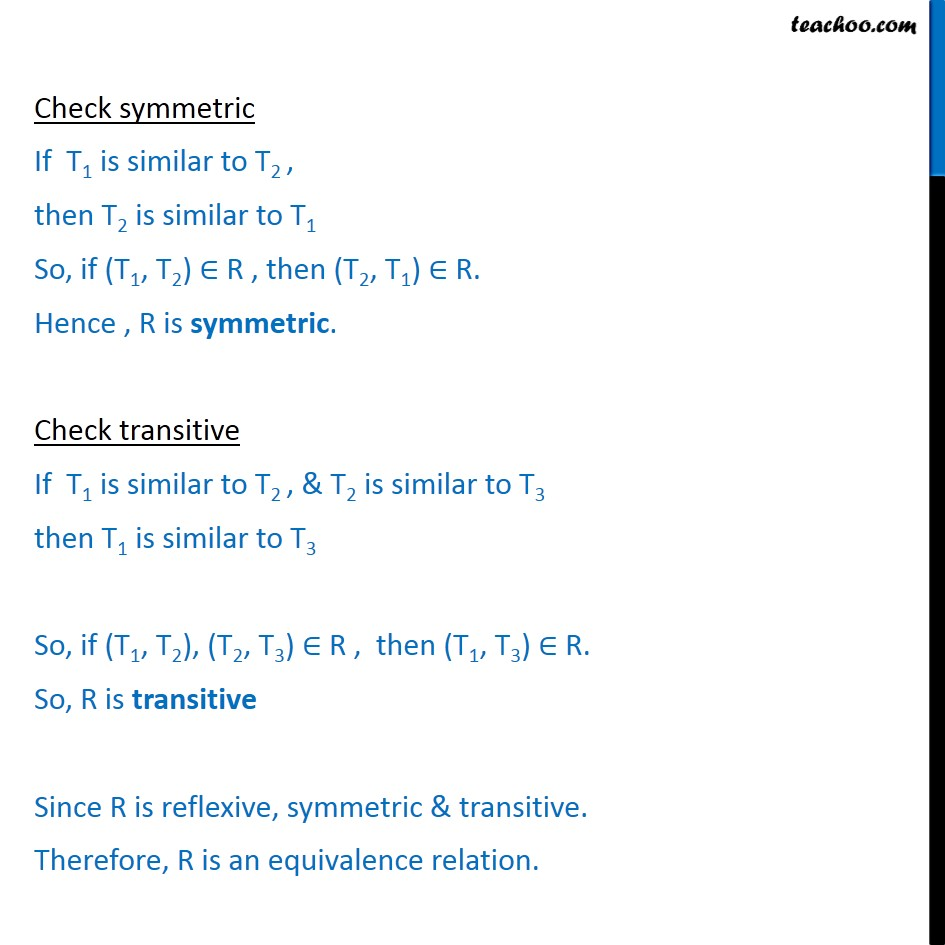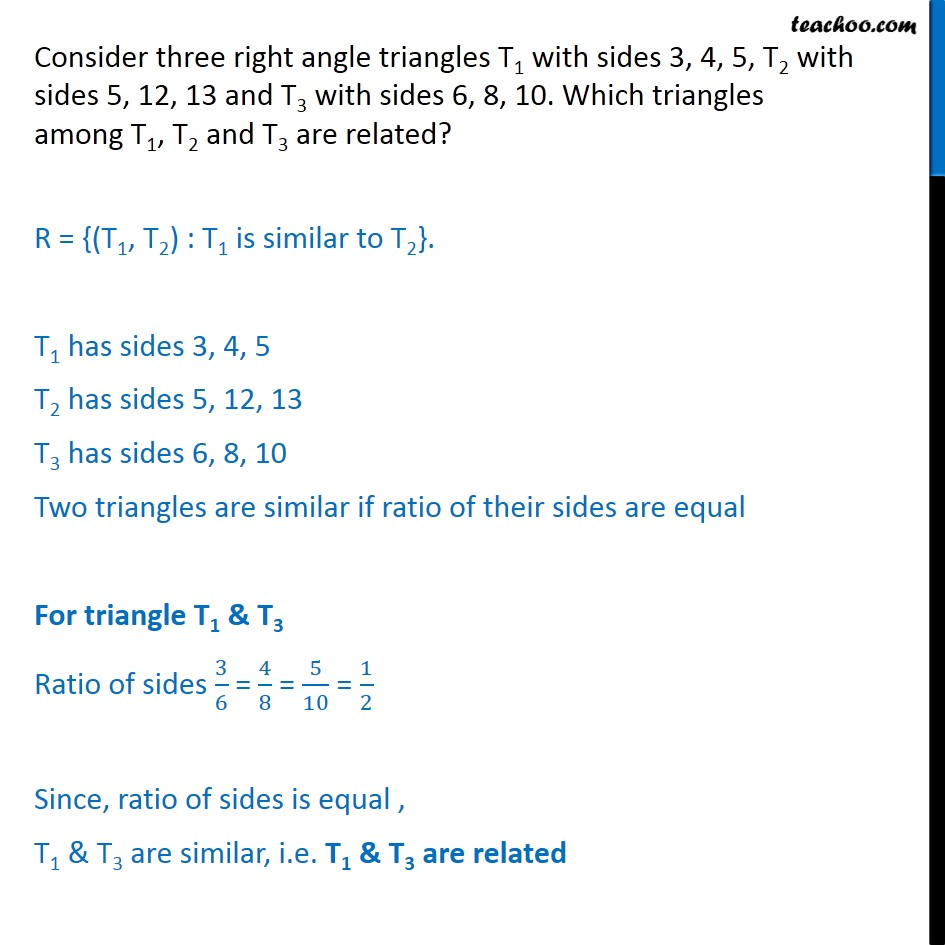1. Chapter 1 Class 12 Relation and Functions
2. Serial order wise
3. Ex 1.1

Transcript

Ex 1.1, 12 Show that the relation R defined in the set A of all triangles as R = {(T1, T2): T1 is similar to T2}, is equivalence relation. Consider three right angle triangles T1 with sides 3, 4, 5, T2 with sides 5, 12, 13 and T3 with sides 6, 8, 10. Which triangles among T1, T2 and T3 are related? R = {(T1, T2) : T1 is similar to T2}. Check reflexive Since every triangle is similar to itself Triangle T is similar to triangle T (T , T) R So, R is reflexive Check symmetric If T1 is similar to T2 , then T2 is similar to T1 So, if (T1, T2) R , then (T2, T1) R. Hence , R is symmetric. Check transitive If T1 is similar to T2 , & T2 is similar to T3 then T1 is similar to T3 So, if (T1, T2), (T2, T3) R , then (T1, T3) R. So, R is transitive Since R is reflexive, symmetric & transitive. Therefore, R is an equivalence relation. Consider three right angle triangles T1 with sides 3, 4, 5, T2 with sides 5, 12, 13 and T3 with sides 6, 8, 10. Which triangles among T1, T2 and T3 are related? R = {(T1, T2) : T1 is similar to T2}. T1 has sides 3, 4, 5 T2 has sides 5, 12, 13 T3 has sides 6, 8, 10 Two triangles are similar if ratio of their sides are equal For triangle T1 & T3 Ratio of sides 3 6 = 4 8 = 5 10 = 1 2 Since, ratio of sides is equal , T1 & T3 are similar, i.e. T1 & T3 are related

Ex 1.1